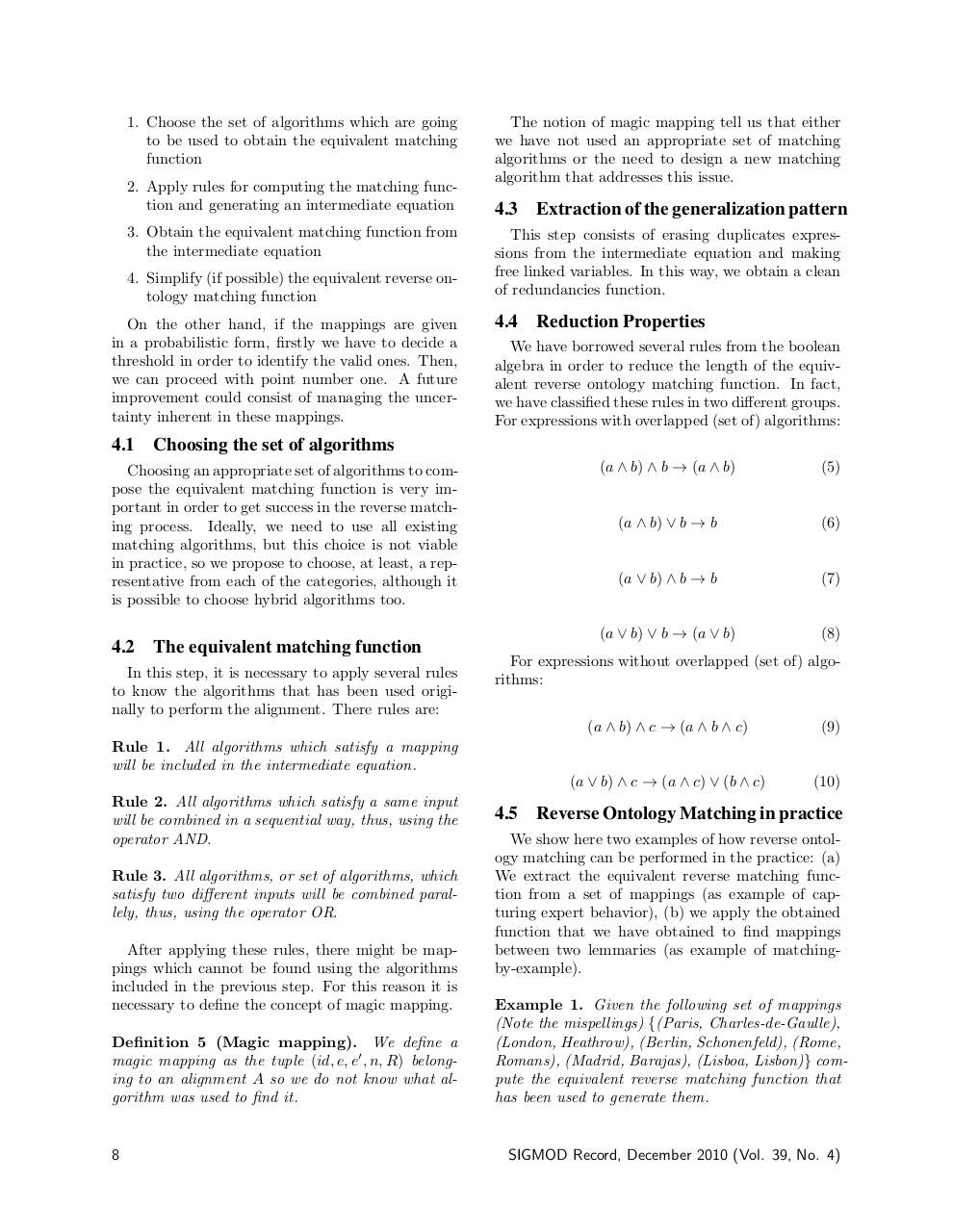# PDF Archive

Easily share your PDF documents with your contacts, on the Web and Social Networks.

## Reverse Ontology Matching.pdfPage 1 2 3 4 5 6 7

#### Text preview

1. Choose the set of algorithms which are going
to be used to obtain the equivalent matching
function
2. Apply rules for computing the matching function and generating an intermediate equation
3. Obtain the equivalent matching function from
the intermediate equation
4. Simplify (if possible) the equivalent reverse ontology matching function
On the other hand, if the mappings are given
in a probabilistic form, firstly we have to decide a
threshold in order to identify the valid ones. Then,
we can proceed with point number one. A future
improvement could consist of managing the uncertainty inherent in these mappings.

4.1

The equivalent matching function

In this step, it is necessary to apply several rules
to know the algorithms that has been used originally to perform the alignment. There rules are:
Rule 1. All algorithms which satisfy a mapping
will be included in the intermediate equation.
Rule 2. All algorithms which satisfy a same input
will be combined in a sequential way, thus, using the
operator AND.
Rule 3. All algorithms, or set of algorithms, which
satisfy two different inputs will be combined parallely, thus, using the operator OR.
After applying these rules, there might be mappings which cannot be found using the algorithms
included in the previous step. For this reason it is
necessary to define the concept of magic mapping.
Definition 5 (Magic mapping). We define a
magic mapping as the tuple (id, e, e0 , n, R) belonging to an alignment A so we do not know what algorithm was used to find it.

8

4.3 Extraction of the generalization pattern
This step consists of erasing duplicates expressions from the intermediate equation and making
free linked variables. In this way, we obtain a clean
of redundancies function.

4.4 Reduction Properties
We have borrowed several rules from the boolean
algebra in order to reduce the length of the equivalent reverse ontology matching function. In fact,
we have classified these rules in two different groups.
For expressions with overlapped (set of) algorithms:

Choosing the set of algorithms

Choosing an appropriate set of algorithms to compose the equivalent matching function is very important in order to get success in the reverse matching process. Ideally, we need to use all existing
matching algorithms, but this choice is not viable
in practice, so we propose to choose, at least, a representative from each of the categories, although it
is possible to choose hybrid algorithms too.

4.2

The notion of magic mapping tell us that either
we have not used an appropriate set of matching
algorithms or the need to design a new matching

(a ∧ b) ∧ b → (a ∧ b)

(5)

(a ∧ b) ∨ b → b

(6)

(a ∨ b) ∧ b → b

(7)

(a ∨ b) ∨ b → (a ∨ b)

(8)

For expressions without overlapped (set of) algorithms:
(a ∧ b) ∧ c → (a ∧ b ∧ c)

(9)

(a ∨ b) ∧ c → (a ∧ c) ∨ (b ∧ c)

(10)

4.5 Reverse Ontology Matching in practice
We show here two examples of how reverse ontology matching can be performed in the practice: (a)
We extract the equivalent reverse matching function from a set of mappings (as example of capturing expert behavior), (b) we apply the obtained
function that we have obtained to find mappings
between two lemmaries (as example of matchingby-example).
Example 1. Given the following set of mappings
(Note the mispellings) {(Paris, Charles-de-Gaulle),
(London, Heathrow), (Berlin, Schonenfeld), (Rome,
Romans), (Madrid, Barajas), (Lisboa, Lisbon)} compute the equivalent reverse matching function that
has been used to generate them.

SIGMOD Record, December 2010 (Vol. 39, No. 4)